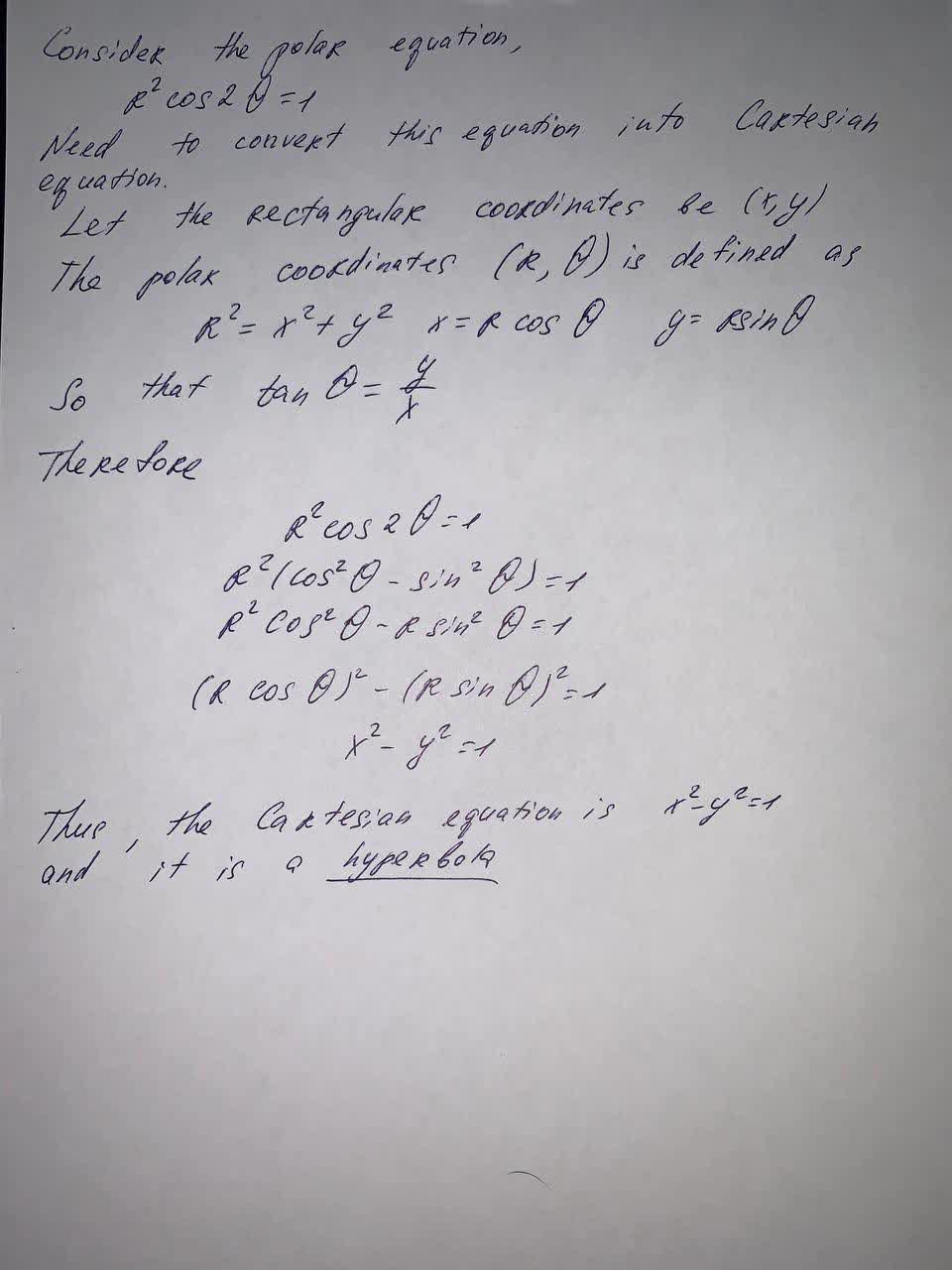Question# Find a Cartesian equation for the curve and identify it. r^2 \cos 2\theta =1

Parametric equations
ANSWEREDFind a Cartesian equation for the curve and identify it.
$$\displaystyle{r}^{{2}}{\cos{{2}}}\theta={1}$$